# Polynomial FactorizationPage 6

#### WATCH ALL SLIDES

Slide 46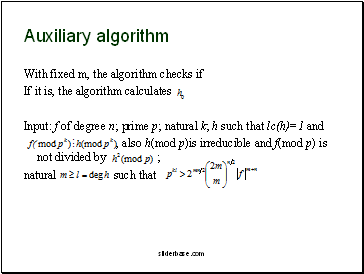## Auxiliary algorith

With fixed m, the algorith checks if

If it is, the algorith calculates

Input: f of degree n; prime p; natural k; h such that lc(h)=1 and

, also h(mod p)is irreducible and f(mod p) is not divided by ;

natural such that

Slide 47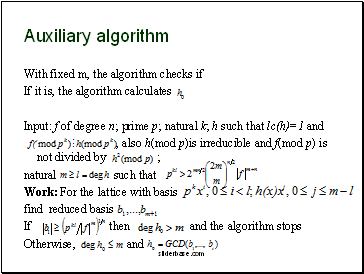Auxiliary algorith

With fixed m, the algorith checks if

If it is, the algorith calculates

Input: f of degree n; prime p; natural k; h such that lc(h)=1 and

, also h(mod p)is irreducible and f(mod p) is not divided by ;

natural such that

Work: For the lattice with basis

find reduced basis

If then and the algorith stops

Otherwise, and

Slide 48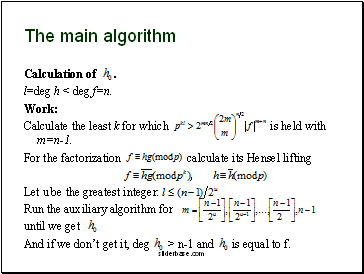## The main algorith

Calculation of .

l=deg h < deg f=n.

Work:

Calculate the least k for which is held with m=n-1.

For the factorization calculate its Hensel lifting

,

Let u be the greatest integer:

Run the auxiliary algorith for

until we get

And if we don’t get it, deg > n-1 and is equal to f.

Slide 49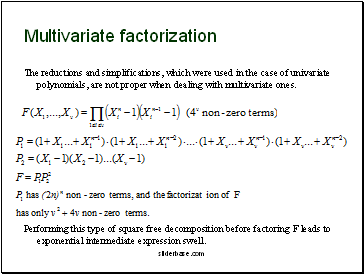## Multivariate factorization

The reductions and simplifications, which were used in the case of univariate polynomials, are not proper when dealing with multivariate ones.

Performing this type of square free decomposition before factoring F leads to exponential intermediate expression swell.

Slide 50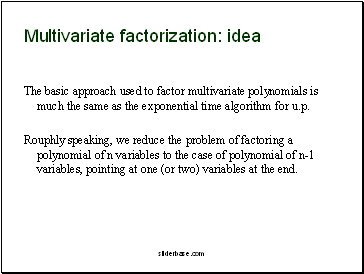Multivariate factorization: idea

The basic approach used to factor multivariate polynomials is much the same as the exponential time algorith for u.p.

Rouphly speaking, we reduce the problem of factoring a polynomial of n variables to the case of polynomial of n-1 variables, pointing at one (or two) variables at the end.

Slide 51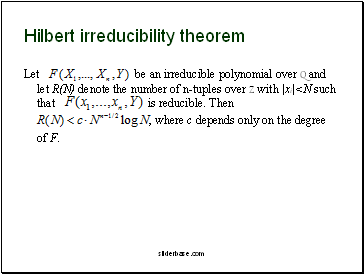## Hilbert irreducibility theorem

Let be an irreducible polynomial over Q and let R(N) denote the number of n-tuples over Z with |xi|<N such that is reducible. Then

, where c depends only on the degree of F.

Slide 52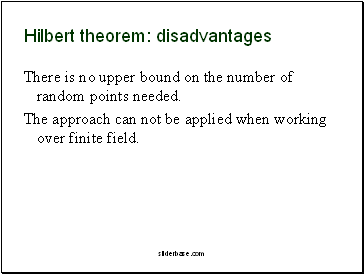There is no upper bound on the number of random points needed.

The approach can not be applied when working over finite field.

Slide 53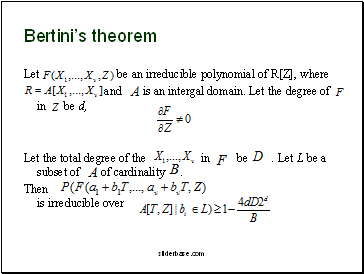## Bertini’s theorem

Let be an irreducible polynomial of R[Z], where

and is an intergal domain. Let the degree of in be d,

Go to page:
1  2  3  4  5  6  7